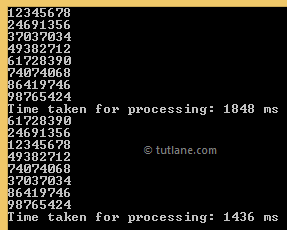# PLINQ (Parallel LINQ)

PLINQ stands for Parallel LINQ. Parallel LINQ (PLINQ) is a parallel implementation of LINQ to Objects. PLINQ supports Parallel programming and is closely related to the Task Parallel Library. In very simple words, PLINQ enables a query to automatically take advantage of multiple processors. PLINQ can significantly increase the speed of LINQ to Objects queries by using all available cores on the host computer more efficiently. This improved performance brings high-performance computing power onto the desktop.

## Syntax of PLINQ

Following is the syntax of using PLINQ to increase the performance of LINQ queries in c#, vb.net.

C# Code

IEnumerable<int> rvals = Enumerable.Range(1, 100000000);
var result1 = rvals.AsParallel().Where(x => x % 12345678 == 0).Select(x => x);

VB.NET Code

Dim rvals As IEnumerable(Of Integer) = Enumerable.Range(1, 100000000)
Dim result1 = rvals.AsParallel().Where(Function(x) x Mod 12345678 = 0).[Select](Function(x) x)

If you observe above syntax we used Parallel method with LINQ queries to increase performance of LINQ queries.

## Example of PLINQ

Following is the example of PLINQ in C# / VB.NET.

C# Code

using System;
using System.Collections.Generic;
using System.Diagnostics;
using System.Linq;

namespace Linqtutorials
{
class Program
{
static void Main(string[] args)
{
// Using LINQ
IEnumerable<int> rvals = Enumerable.Range(1, 100000000);
var result = rvals.Where(x => x % 12345678 == 0).Select(x => x);
Stopwatch sq = Stopwatch.StartNew();
foreach (var item in result)
Console.WriteLine(item);
Console.WriteLine("Time taken for processing: " + sq.ElapsedMilliseconds + "ms");
// Using PLINQ
var result1 = rvals.AsParallel().Where(x => x % 12345678 == 0).Select(x => x);
sq.Restart();
foreach (var item in result1)
Console.WriteLine(item);
Console.WriteLine("Time taken for processing: " + sq.ElapsedMilliseconds + "ms");
}
}
}

VB.NET Code

Module Module1
Sub Main()
Dim rvals As IEnumerable(Of Integer) = Enumerable.Range(1, 100000000)
Dim result = rvals.Where(Function(x) x Mod 12345678 = 0).[Select](Function(x) x)
Dim sq As Stopwatch = Stopwatch.StartNew()
For Each item In result
Console.WriteLine(item)
Next
Console.WriteLine("Time taken for processing: {0} ms", sq.ElapsedMilliseconds)
Dim result1 = rvals.AsParallel().Where(Function(x) x Mod 12345678 = 0).[Select](Function(x) x)
sq.Restart()
For Each item In result1
Console.WriteLine(item)
Next
Console.WriteLine("Time taken for processing: {0} ms", sq.ElapsedMilliseconds)
End Sub
End Module

If you observe the above example, we specified a range of integer numbers starting from “1” to “100000000”. We are dividing numbers in the range to find out numbers that are divided by “12345678” and calculating how much time it takes to finish the division of all numbers for normal LINQ and PLINQ.  Here we used Stopwatch to calculate the time taken to perform this task.

## Result of PLINQ Example

Following is the result of the PLINQ example.This is how we can use PLINQ or Parallel LINQ in c#, vb.net to improve the performance of LINQ queries.